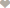Nigeria Student Forum

Welcome, Guest: Join Nigeria Student Forum / Login / Trending Now / Recent

Stats: 17,489 members, 69,475 Topics, 15,463 Comments. Date: Jun 20 2019, 8:07 pm

QUESTION: Simplify The Following Trigonometry [Solved]

Nigeria Student Forum / Questions / Mathematics / Simplify The Following Trigonometry

(Go Down)Answered: Simplify The Following Trigonometry7:50pm on January 11
Jonathan Irhodia

100Level, Nigeria.
For ease of convenience I'll only answer two questions

a. tanΦ - cosΦ
Recall tanΦ = sinΦ+cosΦ
therefore sinΦ+cosΦ - cosΦ = sinΦ
tanΦ - cosΦ = sinΦ

b. tanΦ + cosΦ
Recall tanΦ = sinΦ+cosΦ
therefore sinΦ+cosΦ + cosΦ = sinΦ + 2cosΦ
tanΦ + cosΦ= sinΦ + 2cosΦ2 UpVotes

Simplify The Following Trigonometry8:36pm on January 11
Nofiu RidwanNational open university of Nigeria (Noun)
Bsc. Economic
300Level, Nigeria.
To simplify trigonometric expressions, the calculator uses various formulas of trigonometry, here are some examples of formulas trigonometric used by the calculator:

cos(−x)=cos(x)
sin(−x)=−sin(x)
tan(−x)=−tan(x)
cos(x+π2)=−sin(x)
sin(x+π2)=cos(x)
tan(x+π2)=1tan(x)
cos(x+π)=−cos(x)
sin(x+π)=−sin(x)
tan(x+π)=tan(x)1 UpVote

Full Name:
Email: